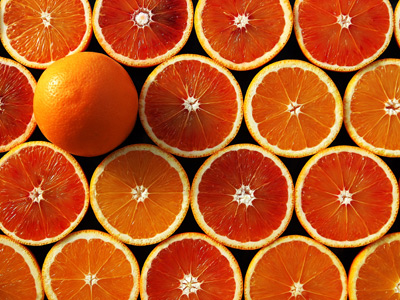Ben has x oranges and Jay has three more oranges than Ben.

# Level 3-4 Algebra - Getting Started

Algebra is a key part of KS3 Maths. But what exactly is it? Well, algebra often substitutes letters for numbers. This is usually to represent a number that we don't yet know.

Most advanced maths relies on algebra so think of this quiz as a springboard into other exciting things! Algebra goes hand in hand with BIDMAS (or BODMAS, they both mean the same thing). You'll need good Algebra skills if you want to do well in science, geography, engineering, or medicine - not just maths. Most of the technological advances we've made over the last 300 years came about due to algebra. And, you can use it in your everyday life too!

At first glance, algebra may seem like a foreign language - all those strange letters and symbols. But it's not too difficult once you understand it. Take your time playing this quiz. Read each question carefully and make sure you understand it before choosing your answer. Once you feel ready, try your hand at this quiz and get started in the exciting world of algebra. Who knows where it might lead you?

1.
Sam has x guinea pigs, Paul has y guinea pigs and Joseph has z guinea pigs. We know that Sam and Paul together have the same number of guinea pigs as Joseph. How would we write this?
x + y = z
xyz
2x + y
x - y = z
Sam's (x) + Paul's (y) = Joseph's (x)
2.
Peter has x oranges and Joe has three more oranges than Peter. How would we write the number of oranges that Joe has?
3x
x + 3
x + x + x + x
x3
3 more is the same as + 3
3.
There are n boys in a football team. How would we write the number of boys in four football teams?
n x n x n x n
4 + n
n + 4
4n
The correct answer means the same as n + n + n + n
4.
Jane has y sweets and Lisa has two sweets less than Jane. How would we write the number of sweets that Lisa has?
2y
-2y
y - 2
y - y - y
2 less is the same as minus 2
5.
Thomas has x marbles and Richard has only one third as many. How would we write the number of marbles that Richard has?
x + 3
x - 3
x / 3
3x
To get one third just divide by 3
6.
Which of these is not an 'equation'?
3x = 7
4x2 - 6 = 28
4x + 5y + 6z
5x = 6y
An 'equation' always contains an = sign
7.
One of the following would never be written - which one?
x + y
1x + y
3x + 2y
4x - 6y
8.
Which of these is a single 'term'?
2a
2a + b2
3a + 2b
3a + b + c
2a is a 'term', the other answers contain more than one 'term'
9.
What is the meaning of 4x
4 divided by x
4 minus x
4 plus x
4 times x
Numbers and letters 'joined together' means multiply them together
10.
Which of these is an 'expression'?
3x
3x + 4y
3x + 4y2
All of the above
An 'expression' contains one or more terms